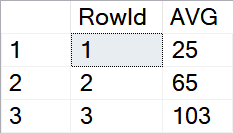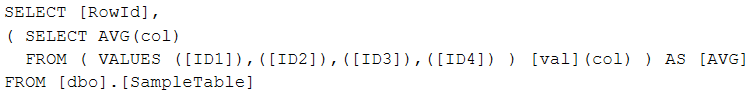T-SQL

# Calculating average value of multiple columns

It’s an easy task to calculate the average AVG() value for all rows in one or more columns. But it starts to be a little bit more interesting when we should calculate the average value for more columns on the same row. There are basically two options on how to do that. One of them is really cool using the row constructor and may be suitable for dynamic SQL because we don’t need to calculate the number of columns involved in the calculation.

```DROP TABLE IF EXISTS [dbo].[SampleTable]

CREATE TABLE [dbo].[SampleTable] (
[RowId] INT NOT NULL IDENTITY PRIMARY KEY,
[ID1] INT NOT NULL,
[ID2] INT NOT NULL,
[ID3] INT NOT NULL,
[ID4] INT NOT NULL
)
GO

INSERT INTO [dbo].[SampleTable]
( [ID1], [ID2], [ID3], [ID4] )
VALUES
( 10, 20, 30, 40 ),
( 50, 60, 70, 80 ),
( 90, 100, 110, 112 )
GO```

First, we will calculate the average traditional way overall values in a column:

```SELECT
AVG([ID1]) [AVG_ID1], AVG([ID2]) [AVG_ID2], AVG([ID3]) [AVG_ID3], AVG([ID4]) [AVG_ID4]
FROM [dbo].[SampleTable]
GO```There is no surprise at all.

But how should we calculate the average value for all columns in a row (except the RowId column)? Sure, we can do it mathematically and explicitly:

```SELECT
[RowId],
([ID1] + [ID2] + [ID3] + [ID4]) / 4 [AVG]
FROM [dbo].[SampleTable]
GO
```What I don’t like about this solution is the constant (4) as the number of columns involved in the calculation. It’s the value we have calculated outside the query itself and then used it inside it.

There is a better solution: We can use the row constructor VALUES() together with the AVG() function to get the same and correct result without any “artificial” constant to be used:

```SELECT
[RowId],
(SELECT AVG([col])
FROM ( VALUES ([ID1]), ([ID2]), ([ID3]), ([ID4]) ) [val] ([col])) AS [AVG]
FROM [dbo].[SampleTable]
GO```

What do you think about it?

It can be used in the case of dynamic SQL effectively without the need to calculate the number of columns involved and handling the constant dynamically:

```DECLARE @Stmt NVARCHAR(MAX)
DECLARE @ColList NVARCHAR(MAX)

SELECT
@ColList = STRING_AGG('([' + [c].[name] + '])', ',' ) WITHIN GROUP (ORDER BY [c].[name])
FROM [sys].[tables] [t]
INNER JOIN [sys].[columns] [c] ON [c].[object_id] = [t].[object_id]
INNER JOIN [sys].[schemas] [s] ON [s].[schema_id] = [t].[schema_id]
WHERE [s].[name] = 'dbo' AND [t].[name] = 'SampleTable' AND c.[name] <> 'RowId'

SET @Stmt = 'SELECT [RowId],' + CHAR(13) +
'( SELECT AVG(col) ' + CHAR(13) +
'  FROM ( VALUES ' + @ColList + ' ) [val](col) ) AS [AVG]' + CHAR(13) +
'FROM [dbo].[SampleTable]'

PRINT @Stmt

EXECUTE(@Stmt)
GO```The VALUES() limitation of a maximum of 1000 rows used at once is overridden there because VALUES() is used in a subquery. You can read more about this problem in this post.

As a next step, it will be interesting to compare both solutions performance-wise on a wide table with let’s say > 100 columns.